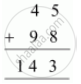Share

# Find the Values of the Letters in the Following and Give Reasons for the Steps Involved. 4 A+ 9 8=C B 3 - Mathematics

Course
ConceptLetters for Digits

#### Question

Find the values of the letters in the following and give reasons for the steps involved.

4 A

+ 9 8

------

C  B 3

------

#### Solution

The addition of A and 8 is giving 3 i.e., a number whose ones digit is 3. This is possible only when digit A is 5. In that case, the addition of A and 8 will give 13 and thus, 1 will be the carry for the next step. In the next step,

1 + 4 + 9 = 14

Therefore, the addition is as follows.Clearly, B and C are 4 and 1 respectively.

Hence, A, B, and C are 5, 4, and 1 respectively.

Is there an error in this question or solution?

#### APPEARS IN

NCERT Solution for Mathematics Textbook for Class 8 (2018 to Current)
Chapter 16: Playing with Numbers
Ex. 16.1 | Q: 2 | Page no. 255

#### Video TutorialsVIEW ALL 

Solution Find the Values of the Letters in the Following and Give Reasons for the Steps Involved. 4 A+ 9 8=C B 3 Concept: Letters for Digits.
S